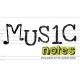# Area of a Triangle – Math Song (Music Video)The area of any given triangle equals one half of the base time the height of that triangle or a=1/2bh. This is such an easy formula to remember and students usually do a great job of saying the formula but students seem to forget that they have to divide by two in order to complete the arithmetic. It’s important that students remember that multiplying by a half is the same as dividing by two. Mr. D clearly explains this in his song “Area of a Triangle.”

Check out more of our song and video resources here: https://squareup.com/market/music-notes-llc

### Join the discussion One Comment

•Dewi says:

Love this, but think my kids would spend the rest of the lesson laughing if I showed them this. Still, if it helps them to remember it, then it works!